# Icing Load and Risk Forecasting for Power Transmission Line Based on Multi-scale Time Series Phase-Space Reconstruction and Regression

Icing Load and Risk Forecasting for Power Transmission Line Based on Multi-scale Time Series Phase-Space Reconstruction and Regression
School of Information, Yunnan University, Kunming 650500, Yunnan, China

Electric Power Research Institute, Yunnan Power Grid Corp, Kunming 650217, Yunnan, China

Corresponding Author Email:
renwenping3053@sina.com
Page:
79-90
|
DOI:
https://doi.org/10.18280/ijsse.110109
19 July 2019
|
Accepted:
11 October 2020
|
Published:
28 February 2021
| Citation

OPEN ACCESS

Abstract:

Keywords:

power transmission line, ensemble empirical mode decomposition, multi-scale; icing alarming; combination forecasting model

1. Introduction

Power transmission line icing could lead to accidents such as insulator icing flashover, wire galloping, disconnections, flashovers, and communication interruptions, which seriously threaten the safe and stable operation of an electric power system [1-4]. To reduce the occurrence of power transmission line icing accident and ensure the safety and reliability of the electric power system operation the accurate prediction of the icing load has become one of the problems that urgently needs to be solved in the construction of smart grids.

At present, the commonly used methods of icing load prediction for power transmission lines include the artificial simulation method, statistical analysis method, Kalman filter method, fuzzy logic method, data-driven method, neural network method, and support vector machine method [5-13]. The artificial simulation method mainly simulates the icing forms of the power transmission lines under different environmental parameters and wires with different diameters in a wind tunnel facility, notably including the Goodwin model , the Makkonen model  and so on. However, the climatic conditions in the artificial simulation are largely different from those in an actual situation. The statistical analysis method establishes a model that is suitable for fitting with the line icing distribution by analysing many years of icing observation data [7, 8]. Yet, its prediction result is obtained based on the icing reappearance period, which cannot predict an accurate icing value. The validity of the Kalman filter method is established on the premise that the characteristics of the noise statistics are known, but this method has certain limitations for power transmission lines with unknown statistical characteristics . Although the fuzzy logic method can establish a linear model that approximates the nonlinear, dynamic, and changing icing thickness, its learning ability is relatively weak . The data-driven method has obtained better prediction results under specific icing scenarios for power transmission lines, but it shows shortcomings of poor learning ability and performance, and its modeling robustness is still lacking . Under large sample conditions, the neural network method has issues such as a long model optimization time, and poor generalization ability. Besides, the single neural network method easily falls into the local optimum, and cannot always reach the expected accuracy . The support vector machine method uses the principle of structural risk minimization, which to a certain extent solves the problem of over-fitting present in the traditional neural network model . However, it still relies on human experience in selecting the kernel function and determining the model parameters.

Each of the above icing prediction methods has its own characteristics, but they each have certain limitations during actual application. Taking into account the nonlinearity, non-stationarity, and intermittency of the icing itself, the traditional single-prediction model can no longer satisfy the requirements of precision. Therefore, the idea of combined prediction is used in connection with the characteristics of each algorithm to establish an icing prediction model making use of complementary advantages, which can improve the prediction precision of the model.

In this paper, the Ensemble Empirical Mode Decomposition (EEMD) and Support Vector Regression (SVR) methods are combined to establish a combined prediction model for the icing load of power transmission lines based on EEMD-SVR. First, EEMD is used to decompose the time series of the icing load into a series of Intrinsic Model Function (IMF) components with different features and a residual component, and the mutual information method and the false nearest neighbor method are used to determine the optimal delay time τ and the embedding dimension m for various IMF components to carry out Phase-Space Reconstruction (PSR) on various subsequences. Then, in connection with the features of each subsequence, different kernel functions are selected, the Particle Swarm Optimization (PSO) algorithm is introduced to optimize and determine the model parameters such as the penalty coefficient, and the SVR prediction model is established. Finally, a value for the icing load is predicted by superimposing the various subsequence prediction results, using fuzzy inference system to determine the risk status of transmission line towers.

The main contributions of this study are demonstrated as follows: (a) A novel icing load prediction model is proposed by combing the EEMD, PSR and SVR; (b) The real performance of the SVR algorithm in the EMD or EEMD based field icing load on power transmission line has not been studied before; (c)PSO algorithms has been established to improve the convergence and iteration speeds and effectively prevent the proposed model and other comparing models; (d) Other eight single or hybrid icing load forecasting model including SVR model, EMD-SVR model, ELM(Extreme Learning Machine) model, EMD-ELM model, EEMD-ELM model, RBF(Radial Basis Function) model, EMD-RBF model and EEMD-RBF model have been compared fully with the proposed model to verify the prediction performance of our proposed model. Through the case analysis of power transmission line icing disasters of the Yunnan Power Grid, the combined model proposed in this paper shows higher prediction precision than the traditional single or hybrid models.

The remainder of this paper is organized as follows: Section 2 briefly reviews the principles of EMD, EEMD, PSR, and SVR. Section 3 details our proposed method with both theoretical analysis and algorithmic description. Section 4 reports our simulation results, and Section 5 concludes this paper with further remarks.

2. Basic Principles

2.1 Principles of EMD and EEMD

EMD is an adaptive data mining method based on signal analysis. By continuously rejecting the mean value of the upper and lower envelopes linked with the maximum value and the minimum value, the original signal is decomposed into several IMF components of different scales and a residual component . The steps for decomposing the time series of the icing load are as follows:

Step 1: Obtain the extremum points of the original signal x(t), and use the spline difference function to fit the upper and lower envelope curves of x(t), which are l1(t) and l2(t), respectively;

Step 2: Obtain the difference between the original signal sequence and the mean value of the envelope curve:

$m_{1}(t)=x(t)-\frac{1}{2}\left(l_{1}(t)+l_{2}(t)\right)$    (1)

Step 3: Judge whether or not m1(t) satisfies the following two conditions: 1) the number of extremum points is equal to or within one of the number of zero-crossing points and 2) the sequence mean value within the domain range approaches zero. If these conditions are not satisfied, then repeat Step 1 and Step 2 until the k-th iteration obtains an m1k(t) that satisfies the conditions, and obtain the IMF1 component:

$C_{1}(t)=m_{1 k}(t)$    (2)

Step 4: Separate the original signal from IMF1, take the residual component r1(t)= x(t)- C1(t) as the original signal, repeat the aforementioned steps, and obtain n IMF components. If the residual component rn(t) is in line with monotonicity, then the decomposition result of x(t) is:

$x(t)=\sum_{i=1}^{n} C_{i}(t)+r_{n}(t)$    (3)

where, Ci(t) is the IMF component, and rn(t) is the residual component.

The monitoring and collection of the icing load for the power transmission lines would often have pulse interference signals that occur due to signal interruption and equipment faults, leading to the appearance of erroneous IMF components in the EMD decomposition process.

EEMD solves the problem of the frequency-mode aliasing that appears in EMD by adding auxiliary noise to eliminate the intermittent phenomenon existing in the original signal . The improvement process is as follows:

Step 1: Add a random Gaussian white noise sequence nm(t) to the original signal x(t) to obtain a new target sequence xm(t):

$x_{m}(t)=x(t)+n_{m}(t)$    (4)

Step 2: Use EMD to decompose xm(t) into n IMF components Cim(t) and one residual component rnm(t).

Step 3: Iterate the above two steps M times in a cycle, each time adding a different white noise sequence with an equal root mean square, and find the mean of the IMF components obtained after M cycles of decomposition as the final decomposition result of EEMD.

2.2 PSR

A relatively stationary subsequence is obtained after performing EEMD, and its stability is enhanced. However, the icing process for the power transmission lines has a nonperiodic motion and stronger sensitivity. In reference , the chaotic characteristics of the time series of the icing load are verified by calculating the largest Lyapunov exponent. The PSR method is used in this paper to analyze the intrinsic attributes of icing load changes.

PSR is the basis for the application of nonlinear dynamics theory, which can transform the one-dimensional time series of the icing load into a multidimensional phase space. The steps for phase-space reconstruction on various subsequences of the icing load are as follows:

Step 1: Define the time series of the icing load as {xi, i=1,2,…,N}, wherein N is the length of the series.

Step 2: Given the embedding dimension m (m≥2d+1, d is the system dynamics dimension) and τ as the time delay.

Step 3: According to the embedding theorem proposed by Takens, the reconstructed phase space is:

$\left\{x_{i}, x_{i+\tau}, x_{i+(m-1) \tau}\right\}, i=1,2, \ldots, M$    (5)

where, M=N-(m-1)τ is the number of phase points.

In the reconstructed phase space, the selection of the time delay τ and the embedding dimension m directly affects the acquisition of information after the reconstruction. From reference , if τ is too small, it may not be able to show the dynamic features of the system, and if τ is too large, then it can make the simple track complicated and reduce the number of valid data points. Similarly, a too small m embedded in space cannot accommodate the attractors of the dynamic system, and the dynamic characteristics of the system will not be comprehensively reflected, and a too large m can not only reduce the available data length and increase the calculation workload but also increase the prediction error. To determine the appropriate parameters, the mutual information method and the false nearest neighbor method are used in this paper to determine the delay time τ and the embedding dimension m, respectively, of the IMF components.

2.3 SVR principles

The core idea of SVR is to define a nonlinear mapping $\Phi(x)$ based on the principle of structural risk minimization. Map an input dataset to a high-dimensional feature space F. In this high-dimensional feature space, there is a linear function f(x) that can clearly represent the nonlinear relationship between the input dataset and the output dataset, as illustrated in Figure 1, its function is defined as follows [18, 19]:

Given a training set T={(xi,yi), i=1,2,…,l}, wherein $x_{i} \in \boldsymbol{R}^{N}, y_{i} \in \boldsymbol{R}$, construct a linear regression function f(x) in the high-dimensional space F as shown in Formula (4):

$f(x)=\boldsymbol{W}^{T} \Phi(x)+b$    (6)

W and b are the weight vector and the bias, respectively. Introduce the Lagrange multipliers $\delta_{i}^{*}$, $\mu_{i}^{*}$, $\alpha_{i}^{*}$, and ς, and then the target function of the SVR quadratic programming problem is as shown in Formula (5):

\begin{aligned} L_{D}=& \frac{1}{2} \sum_{i=1}^{l} \sum_{i=1}^{l} Q_{i j}\left(\alpha_{i}-\alpha_{i}^{*}\right)\left(\alpha_{j}-\alpha_{j}^{*}\right)+\varepsilon \sum_{i=1}^{l}\left(\alpha_{i}+\alpha_{i}^{*}\right) \\ &-\sum_{i=1}^{l} y_{i}\left(\alpha_{i}-\alpha_{i}^{*}\right)-\sum_{i=1}^{l}\left(\delta_{i} \alpha_{i}+\delta_{i}^{*} \alpha_{i}^{*}\right) \\ &+\sum_{i=1}^{l}\left[\mu_{i}\left(\alpha_{i}-C\right)+\mu_{i}^{*}\left(\alpha_{i}^{*}-C\right)\right]+\varsigma \sum_{i=1}^{l}\left(\alpha_{i}-\alpha_{i}^{*}\right) \end{aligned}    (7)

where, C is the penalty coefficient, $\mathcal{E}$ is the insensitive loss coefficient, and $Q_{i j}=K\left(x_{i}, x_{j}\right)=\Phi^{T}\left(x_{i}\right) \Phi\left(x_{j}\right)$ is the kernel function. Commonly used kernel functions include the linear kernel function, $K\left(x_{i}, x_{j}\right)=x_{i}^{\mathrm{T}} x_{j}$, and the radial basis function (RBF) kernel function, $K\left(x, x_{i}\right)=\exp \left(-\frac{\left\|x-x_{i}\right\|^{2}}{\delta^{2}}\right)$. The regression function can be obtained by solving Formula (5), as shown in Formula (6):

$f(x)=\sum_{i=1}^{l}\left(\alpha_{i}-\alpha_{i}^{*}\right) K\left(x_{i}, x_{j}\right)+b^{*}$    (8)

For sample xi, define an edge function as shown in formula (7):

$h\left(x_{i}\right) \equiv f\left(x_{i}\right)-y_{i}=\sum_{j=1}^{l} Q_{i j} \theta_{j}-y_{i}+b$    (9)

The penalty coefficient C plays a very important role in the complexity and stability of the model. The RBF kernel parameter σ determines the width of the insensitive region of the regression function to the sample data [20, 21]. Therefore, in this paper, optimization is sought between the penalty coefficient C and the kernel parameter σ based on the PSO algorithm. In addition, a mean squared error (MSE) that can reflect the regression performance of the SVR is selected as the fitness function, as shown in Formula (8):

$M S E=\sqrt{\sum_{i=1}^{n} \frac{\hat{y}-y_{i}}{n}}$    (10)

where, $\hat{y}$ is the predicted value of the icing load, yi is the actual value of the icing load, and n is the sample size of the test set.

## 1.png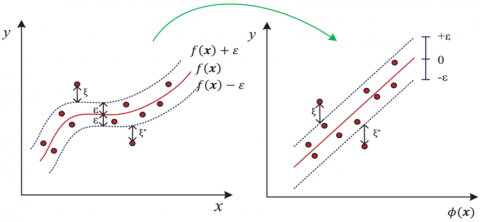Figure 1. Illustration of nonlinear mapping from the input space to a high-dimensional feature space

3. A Combined Prediction Model for the Icing Load Based on EEMD-SVR

The icing load data for power transmission lines are characterized by a large fluctuation range, nonlinearity, non-stationarity, and randomness, which seriously hinder the establishment of a prediction model and has a relatively large effect on the prediction precision. The use of EEMD to separate the various components in the time series of the icing load weakens the non-stationarity of the signal, and then PSR is carried out for each component and SVR is used for modeling and prediction. Finally, the predicted values of the various components are linearly superimposed to obtain the predicted value of the icing load. Figure 2 is a flowchart of the combined prediction model for the icing load of the power transmission lines based on EEMD and SVR put forward in this paper. The specific modeling steps are as follows:

Step 1: Carry out preprocessing on the icing load data set, including outlier extraction, data normalization, and so on;

Step 2: Use the EEMD algorithm to decompose the time series for the icing load to obtain the subsequence components (IMF1 to IMFn) and the residual component rn(t);

Step 3: Use the mutual information method and the false nearest neighbor method to determine the optimal delay time τ and the embedding dimension m, respectively, of the various IMF components, and reconstruct the phase space for various subsequences;

Step 4: Separately establish an SVR model for different reconstructed IMF subsequences, select the optimal kernel function, and use the PSO algorithm to optimize and select the model parameters;

Step 5: Superimpose the prediction result of each subsequence to obtain the predicted value of the icing load;

Step 6: Compare with the actual icing load, calculate the error index, and carry out error analysis.

3.1 Preprocessing of sample data

To reduce the interference of abnormal data with the regression performance and accelerate the training speed and convergence speed of the prediction model, the min-max normalization method is used in this paper to carry out normalization processing on the sample set.

$x_{g}=\left(x-x_{\min }\right) /\left(x_{\max }-x_{\min }\right)$    (11)

where, xg is the data after normalization, x is the original data in the sample set, and xmin and xmax are, respectively, the minimum value and the maximum value of x.

3.2 PSR parameter setting

After EMD or EEMD decomposition is carried out for the time series of the icing load, a series of IMF components with different scales and a residual component are generated, and the time series of the icing load becomes stationary. PSR can extract and recover the variation rule of the original icing load from the time series. Moreover, in PSR, the selection of the time delay τ and the embedding dimension m determines the degree of similarity of the reconstructed phase space with the original system.

The main selection methods for the time delay are the autocorrelation function method and the mutual information method. The autocorrelation function method is mainly suitable for use in linear and small dataset problems, and it can only extract a linear correlation of the time series space. The mutual information method is suitable for use in nonlinear time series. Therefore, the time corresponding to the first time the mutual information function reaches the minimum value point is selected in this paper as the delay time of PSR. For the time series of the icing load {xi, i=1,2,…,N}, set the delay time as τ, and then the time series changes to {xi, i=1,2,…,N}. Let P(xk) be the probability that xk appears in the original time series {xi, i=1,2,…,N}, P(xk) be the probability that xk appears in the time series {xi, i=1,2,…,N} after a delay of τ, and P(xk, xk) be the probability that xk and xk appear together in the two series, and then the mutual information function can be expressed as:

$I(\tau)=\sum_{k=i}^{N} P\left(x_{k}, x_{k+\tau}\right) \log \frac{P\left(x_{k}, x_{k+\tau}\right)}{P\left(x_{k}\right) P\left(x_{k+\tau}\right)}$    (12)

where, the optimal delay time τ is the first minimum value of the mutual information function.

## 2.png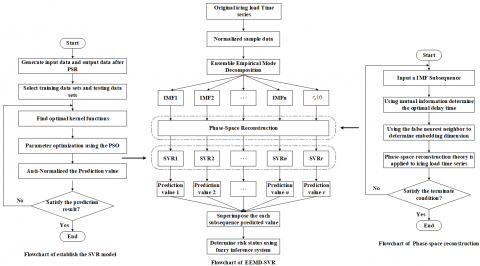Figure 2. Flowchart of icing load forecasting based on EEMD-PSR-SVR

The basic principle of the false nearest neighbor method is that as the embedding dimension m increases, the track of chaotic motion could be gradually opened. The false nearest neighbor points (two points that are originally not adjacent to each other that become adjacent to each other after projection) could be gradually removed, and the original chaotic motion trajectory could be gradually restored. The determination method for the embedding dimension by the false nearest neighbor method is to continuously increase the embedding dimension m starting from 2, calculate the proportion of false nearest neighbor points, and increase the embedding dimension until the proportion of false nearest neighbor points is less than 5% or not changing. The corresponding embedding dimension is the value that is sought.

PSR can expand the subsequence after the EEMD decomposition to N-(m-1)τ samples, wherein there are m input dimensions and one output dimension. In this paper, the SVR model is used to predict the various subsequences after reconstruction to obtain the final prediction result for the icing load.

3.3 SVR model

In this paper, SVR is used to establish a prediction model for the subsequences of the icing load. Different kernel functions are used in connection with different decomposition sequences, and the PSO algorithm is introduced to optimize and select the model parameters. The specific steps are as follows:

Step 1: Select the appropriate training samples and test samples from the data after PSR;

Step 2: Select different kernel functions to test the training data. An RBF kernel function with a strong local adaptive capability can be used in connection with subsequences with obviously greater fluctuations, and a linear kernel function is selected for training subsequences with relatively small fluctuations.

Step 3: Use the PSO algorithm to find the optimal parameters in connection with different training kernel functions. A model that uses linear kernel functions for training only needs to optimize and select the penalty coefficient C, while a model that uses the RBF kernel function for training needs to simultaneously optimize and determine C and the insensitive loss coefficient σ;

Step 4: Use the selected kernel function and the model parameters to establish a regression model for the training data. Input the prediction data, and test the prediction result. If the prediction result is not in line with the prediction precision, then go back to Step 2;

Step 5: After the kernel function and the related parameters are optimized and determined, input the subsequence prediction data to carry out prediction and error analysis;

Step 6: Superimpose the predicted value obtained by each subsequence to obtain the final prediction result for the icing load.

4. Case Analysis

4.1 Data source and evaluation indexes

In this paper, algorithm verification was carried out using the real-time icing loads collected by the online monitoring system, “Tao Luo Xiong”, which is used in the power transmission lines of the Yunnan Power Grid located in northeast Yunnan, which has 2872 data points corresponding to the intermittent ice-accretion processes, and a sampling interval of 15 minutes. The time series of the icing load is shown in Figure 3. The first 2,021 data sample points were selected as the model training set, and data sample points 2,022-2,160 were the test set. The descriptive statistics of the icing load data are shown in Table 1.

The following three indicators were used to evaluate the regression performance of the model:

(1) Mean Absolute Error (MAE)

$M A E=\frac{1}{n} \sum_{i=1}^{n}\left|\hat{y}-y_{i}\right|$    (13)

(2) Mean Relative Error (MRE)

$M R E=\frac{1}{n} \sum_{i=1}^{n}\left|\hat{y}-y_{i}\right| / \operatorname{Mean}\left(y_{i}\right)$    (14)

(3) Root Mean Square Error (RMSE)

$R M S E=\sqrt{\frac{1}{n} \sum_{i=1}^{n}\left|\hat{y}-y_{i}\right|^{2}}$    (15)

where, $\hat{y}$ is the predicted value of icing, yi is the actual value of icing, and n is the sample size of the test set.

## 3.png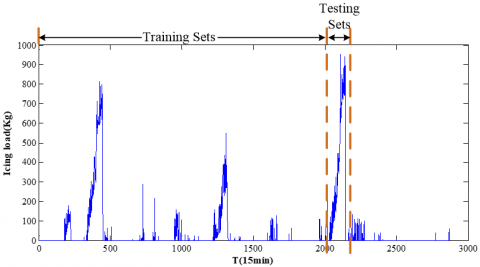Figure 3. Time series of the icing load for the Tao Luo Xiong line

Table 1. Descriptive statistics of the icing load data. (unit:kg)

 Data Min Max Mean SD Icing load 0 951.9 40.23 133.75

4.2 Mode decomposition and optimization of the parameters

The EMD was carried out on the time series of the icing load for the power transmission lines in the field shown in Figure 3 to obtain 13 IMF components (IMF1-IMF13) and one residual component r13(t), as shown in Figure 4(a), and EEMD was carried out to obtain 10 IMF components (IMF1-IMF10) and one residual component r10(t), as shown in Figure 4(b). Comparing Figure 3, Figure 4(a) and Figure 4(b), the intrinsic model components after the EMD or EEMD decomposition are obviously more stationary than those of the original time series of the icing load, and the IMF components sequentially characterize the frequency spectrum features of the original series from high frequency to low frequency.

The mutual information method and the false nearest neighbor method were used to determine the delay time τ and the embedding dimension m of the various IMF components, and the PSO algorithm was introduced to find the optimal parameters for our proposed model and other comparing models of each subsequence, including the C and δ of SVM model, hidden layer nodes L, n of ELM model and RBF model, respectively. See Table 2 and Table 3 for the optimal values of the parameters for each model of subsequence component obtained for the EMD decomposition and EEMD decomposition, respectively.

4.3 Prediction results for the icing load

SVR models were separately established to carry out the prediction in accordance with the characteristics of the different IMF components. From the subsequences obtained by the EEMD decomposition, one can see that the intrinsic model components IMF1-IMF4 had a high fluctuation frequency and relatively high complexity. After multiple model trainings, the RBF kernel function was selected as the kernel function for the model training. Components IMF5-IMF10 and the residual component were more stationary, and a linear kernel function was selected to carry out the prediction. Sigmoid function was selected as the kernel function for the combined ELM Models for training.

## 4.png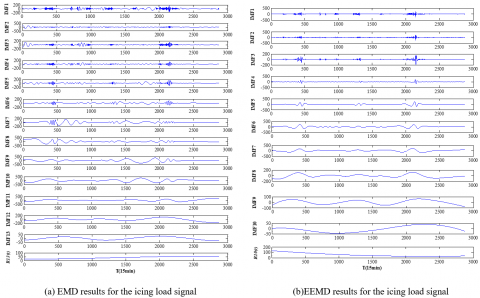Figure 4. EMD and EEMD results for the icing load signal

Table 2. EMD combined model parameters of each subsequence

 Subsequence PSR EMD-SVR EMD-ELM EMD-RBF m τ C σ L n IMF1 4 15 70.7729 4.3572 3 45 IMF2 4 6 18.5735 2.7742 21 27 IMF3 4 11 2.642 7.3416 14 51 IMF4 4 14 62.6944 2.2978 25 39 IMF5 4 17 2.0232 - 11 43 IMF6 4 17 10.5193 - 21 61 IMF7 3 23 99.8593 - 7 74 IMF8 3 25 11.1958 - 38 10 IMF9 3 22 15.1366 - 21 18 IMF10 2 17 7.9203 - 6 17 IMF11 2 15 10.3403 - 12 10 IMF12 2 20 28.4332 - 9 19 IMF13 2 7 57.6768 - 12 83 R13(t) 1 9 36.3223 - 6 44

Note: “-” indicates that the subsequence prediction model does not have this parameter value

Table 3. EEMD combined model parameters of each subsequence

 Subsequence PSR EEMD-SVR EEMD-ELM EEMD-RBF m τ C σ L n IMF1 4 11 5.1455 6.6155 3 39 IMF2 5 2 11.7762 4.2876 7 96 IMF3 6 4 16.4824 3.1952 8 47 IMF4 5 9 7.9869 2.2978 21 49 IMF5 4 17 74.3221 - 17 54 IMF6 3 27 5.6235 - 18 27 IMF7 2 20 5.0396 - 7 34 IMF8 2 23 19.7338 - 13 14 IMF9 2 17 2.7042 - 7 8 IMF10 2 7 4.2876 - 48 59 R10(t) 1 10 7.3518 - 52 7

Note: “-” indicates that the subsequence prediction model does not have this parameter value

## 5.png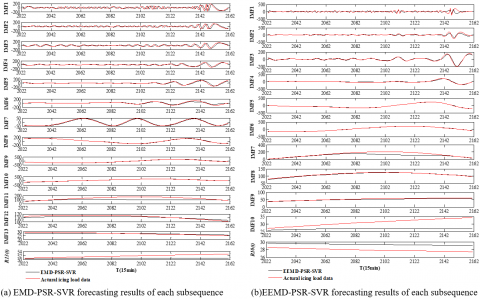Figure 5. EMD-PSR-SVR and EEMD-PSR-SVR forecasting results of each subsequence

Table 4. Evaluation indexes for icing load of nine models

 Prediction Model Evaluation Indexes MAE MRE RMSE RBF 68.3524 22.8% 151.3562 ELM 62.7352 24.5% 125.4293 SVR 56.9396 17.7% 103.6935 EMD-PSR-RBF 55.7536 17.3% 94.9527 EMD-PSR-ELM 58.0701 18.1% 97.0145 EMD-PSR-SVR 52.9575 16.5% 93.4633 EEMD-PSR-RBF 48.1368 14.9% 70.5701 EEMD-PSR-ELM 50.2635 15.3% 78.3392 Proposed model 44.2635 13.7% 66.3392

## 6a.png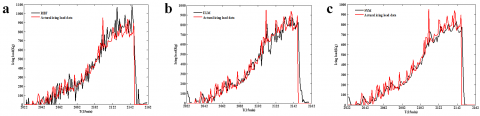## 6b.png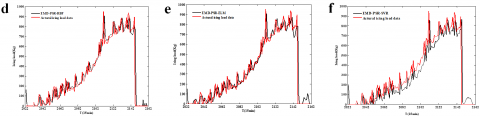## 6c.png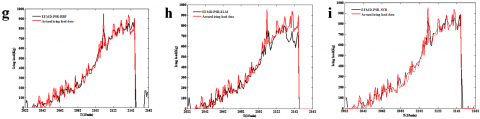Figure 6. Comparison of results among nine icing load forecasting models: (a) RBF, (b) ELM, (c) SVR, (d) EMD-PSR-RBF, (e) EMD-PSR-ELM, (f) EMD-PSR-SVR, (g) EEMD-PSR-RBF, (h) EEMD-PSR-ELM, (i) Proposed model

## 7a.png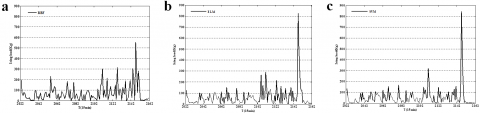## 7b.png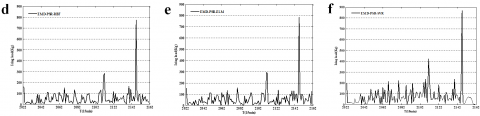## 7c.pngFigure 7. Comparison of absolute curves of error among nine icing load forecasting models: (a)RBF, (b) ELM, (c) SVR, (d) EMD-PSR-RBF, (e) EMD-PSR-ELM, (f) EMD-PSR-SVR, (g) EEMD-PSR-RBF, (h) EEMD-PSR-ELM, (i) Proposed model

Finally, the prediction results of the various IMF components were superimposed to obtain the predicted value of the icing load. To verify the validity and robustness of the model in this paper, RBF model, ELM model, SVR model EMD-RBF model, EEMD-RBF model, EMD-ELM model, EEMD-ELM model and an EMD-SVR model were established to carry out the prediction of the icing load and to calculate the MAE, MRE, and RMSE of each model. The prediction results for the various subsequences of EMD-PSR-SVR model and EEMD-PSR-SVR model in this paper are shown in Figure 5(a) and Figure 5(b), respectively. The prediction results for the icing load using the nine models and the error curve are shown in Figure 6 and Figure 7, respectively. See Table 4 for the error indicators of nine icing load prediction models.

Comparing the prediction error evaluation indexes for the icing load in Table 4 and the icing load prediction curves in Figure 6 and the absolute curves of error in Figure 7, it is easy to see that:

1) All nine models can accurately predict the increasing and decreasing trends of the icing load for the power transmission lines in the field;

2) Compared to the traditional SVR model, the EMD-SVR model and the model of this paper can effectively improve on the precision of the prediction model by performing the mode decomposition of the time series waveforms. In doing so, the MAE decreased by 6.99% and 22.3%, respectively; the MRE decreased by 1.2% and 3.94%, respectively; and the RMSE decreased by 9.86% and 36.1%, respectively.

3) In comparison with the EMD combined model, it can be found that the model of this paper effectively avoided the interference with the waveform decomposition generated by the aliasing phenomenon that appears in the traditional EMD algorithm, thereby verifying the reasonableness of the model of this paper.

4) Due to its real-time nature and accuracy, the model in this paper can effectively carry out the fitting and tracking of the icing load of power transmission lines in the field and react in a timely manner when the icing load is high, which proves the validity of the combined prediction model proposed in this paper.

4.4 Evaluate risk status using fuzzy inference system

For overhead power transmission lines, wind and ice storms may be treated as persistent design situations that have a decisive effect on the reliability of concrete poles and steel towers. Their safety and the same optimal solution are closely related to extreme action effects caused by wind storm pressures on the ice-free and ice-covered surfaces of structures and conductors [22, 23]. The fuzzy inference system is the actual process of mapping from a given input to an output by the fuzzy logic , which utilized to formalize reasoning and take a decision to exhibit the safety of transmission line towers in our study. The membership function is the core of fuzzy inference system, which reflect the gradualism, stability and continuity of the fuzzy concept, and a complete membership function is taken as a convex F set. The commonly used methods to determine the membership function are binary comparison sorting method, fuzzy statistics method, neural network method and expert experience method. For the convenience of calculation and processing, the membership functions obtained by different methods are often approximated into familiar analytic functions, such as triangle, bell, trapezoid, gaussian and Sigmoid. In this paper, the triangle function is utilized as the membership function and shown in Figure 8, the l1, l2, l3, l4, and l5 are icing load of five risk status of transmission line towers, which are very safe, safe, average, dangerous and very dangerous, respectively.

## 8.png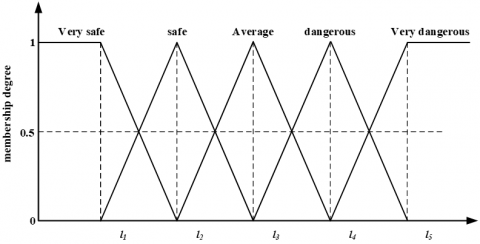Figure 8. The membership function between icing load and risk status

Table 5. The functions and variables utilized in fuzzy logic controller

 Patent block Class Fuzzy logic controller Struct Type Mamdani And method Min Or method Max Defuzz method Centroid Implication method Min Aggregation method Max

Table 6. Risk status of prediction of testing set sample

 Point of test set Range of prediction result(kg) Risk status 2022-2076, 2146-2160 0-177.9 Very safe 2077-2089 189.8-279.3 Safe 2090-2106 315.7-594.1 Average 2107-2120 637.1-819.6 Dangerous 2121-2145 826.1-951.9 Very dangerous

A fuzzy logic controller is used to a design variables to exhibit the risk status of transmission line towers during the icing load of power transmission line under different icing loading conditions, and the prediction result of EEMD-PSR-SVR model and the risk status of transmission line towers as input and output of fuzzy inference system, respectively. The flowchart of evaluate risk status based on fuzzy inference system is shown in Figure 9.

In this study, the range of icing load of the “Tao Luo Xiong” transmission line must be equal or less than 1200kg, otherwise greater than the limits may damage the equipment or device on whole transmission line, and there are five risk status of transmission line towers [25-27]. The functions and variables utilized in fuzzy logic controller are listed in Table 5. The input and output membership functions are drawn by triangular membership functions and shown in Figure 10. Therefore, the icing load of power transmission line range from 830 to 1200kg have been selected as the very dangerous that need deicing and maintenance decisions instantly, and other are represented accordingly by very safe, safe, average and dangerous. The risk status of prediction of testing set sample points 2,022-2,160 as listed in Table 6.

## 9.png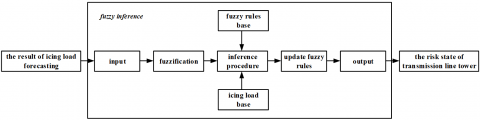Figure 9. Flowchart of evaluate risk status based on fuzzy inference system

## 10.png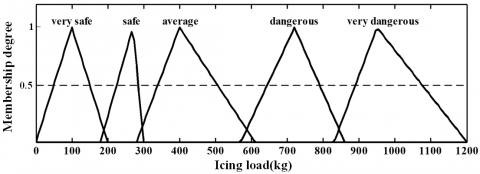Figure 10. Icing load of power transmission line and risk status membership functions

5. Conclusion

A prediction model for the icing load of power transmission lines to ensure the security and reliability of the power grid was established based on EEMD, PSR and SVR that decomposed the time series signals of the original icing load into more stationary waveforms. The PSO algorithm, mutual information method, and false nearest neighbor method were introduced to determine the optimal SVR and PSR parameters. Compared to the other eight traditional icing load prediction model including SVR model, EMD-SVR model, ELM model, EMD-ELM model, EEMD-ELM model, RBF model, EMD-RBF model and EEMD-RBF model, the following conclusions were obtained:

(1) The selection of values for the model parameters and the PSR parameters directly affects the prediction precision. The use of optimization algorithms such as PSO can improve the convergence and iteration speeds and effectively prevent the model from falling into local extremum values;

(2) PSR can better mine the nonlinear dynamic characteristics of the time series of the icing load and can reduce the effect of the non-stationarity and sensitivity present in the original time series of the icing load on the prediction results;

(3) A combined prediction model can effectively improve the prediction precision. Both the EMD and EEMD combined model obtained better prediction results lower prediction error than the single model;

(4) Utilizing fuzzy inference system to determine the risk status of transmission line towers for making deicing and maintenance decisions instantly;

(5) The example shows that the model of this paper can better track the variation rule of icing in the field. In regions with frequent power transmission line icing, the application of this prediction model can better achieve the real-time prediction of icing loads.

Acknowledgment

This study was supported in part by the National Natural Science Foundation of China (NSFC) (61763049) and the Science and Technology plan of Applied Basic Research Programs key Foundation of Yunnan province (2018FA032).

References

 Jiang, X., Zhang Z., Hu, Q., Hu, J., Shu, L. (2018). Thinkings on the restrike of ice and snow disaster to the power grid. High Voltage Engineering, 44: 463-469. https://doi.org/10.13336/j.1003-6520.hve.20180131017

 Li, L., Yang, L., Hao, Y. (2012). Review of on-line monitoring of ice coating on overhead transmission line. Power System Technology, 36: 237-243. https://doi.org/10.13335/j.1000-3673.pst.2012.02.044

 Jiang, X., Xiang, Z., Zhang, Z., Hu, J., Hu, Q., Shu, L. (2014). Predictive model for equivalent ice thickness load on overhead transmission lines based on measured insulator string deviations. IEEE Transactions on Power Delivery, 29(4): 1659-1665.  https://doi.org/10.1109/tpwrd.2014.2305980

 Farzaneh, M., Chisholm, W.A. (2014). 50 years in icing performance of outdoor insulators. IEEE Electrical Insulation Magazine, 30(1): 14-24.  https://doi.org/10.1109/mei.2014.6701103

 Goodwin, E., Mozer, J., DiGioia, A. (1983). Predicting ice and snow loads for transmission line design, predicting ice & snow loads for transmission line design.

 Makkonen, L. (1998). Modeling power line icing in freezing precipitation. Atmospheric Research, 46(1-2): 131-142.  https://doi.org/10.1016/s0169-8095(97)00056-2

 Wang, X., Dai, D., Hao, Y. (2012). Statistics and analysis of transmission lines icing data based on online monitoring system. High Voltage Engineering, 38: 3000-3007. https://doi.org/1003-6520 (2012) 11-3000-08

 Wang, J., Su, S., Sheng, X. (2015). Comparative study of applicability of methods for estimating transmission line icing return period based on various extreme value distributions. Power System Technology, 39: 2614-2620. https://doi.org/10.13335/j.1000-3673.pst.2015.09.036

 Huang, X., Li, H., Zhu, Y. (2017). Short-term forecast for transmission line icing by time series analysis and Kalman filtering. High Voltage Engineering, 43: 1943-1949. https://doi.org/10.13336/j.1003-6520.hve.20170527027

 Huang, X., Wang, Y., Zhu Y. (2016). Icing forecast of transmission line based on genetic algorithm and fuzzy logic. High Voltage Engineering, 42: 1228-1235. https://doi.org/10.13336/j.1003-6520.hve.20160405029

 Huang, X., Xu J., Yang, C. (2014). Transmission line icing prediction based on data driven algorithm and LS-SVM. Automation of Electric Power Systems, 38: 81-86. https://doi.org/10.7500/AEPS20131022011

 Ma, T., Niu, D. (2016). Icing forecasting of high voltage transmission line using weighted least square support vector machine with fireworks algorithm for feature selection. Applied Sciences-Basel, 6(12). https://doi.org/10.3390/app6120438

 Dai, D., Huang, X., Dai, Z. (2013). Regression model for transmission lines icing based on support vector machine. High Voltage Engineering, 39: 2822-2828. https://doi.org/10.3969/j.issn.1003-6520.2013.11.034

 Fan, G.F., Peng, L.L., Hong, W.C., Sun, F. (2016). Electric load forecasting by the SVR model with differential empirical mode decomposition and auto regression. Neurocomputing, 173: 958-970. https://doi.org/10.1016/j.neucom.2015.08.051

 Sun, W., Wang, Y. (2018). Short-term wind speed forecasting based on fast ensemble empirical mode decomposition, phase space reconstruction, sample entropy and improved back-propagation neural network. Energy Conversion and Management, 157: 1-12. https://doi.org/10.1016/j.enconman.2017.11.067

 Li, J., Li, P., Miao, A., Chen, Y., Cao, M., Shen, X. (2018). Online prediction method of icing of overhead power lines based on support vector regression. International Transactions on Electrical Energy Systems, 28(3). https://doi.org/10.1002/etep.2500

 Ghorbani, M.A., Kisi, O., Aalinezhad, M. (2010). A probe into the chaotic nature of daily streamflow time series by correlation dimension and largest Lyapunov methods. Applied Mathematical Modelling, 34(12): 4050-4057. https://doi.org/10.1016/j.apm.2010.03.036

 Cherkassky, V. (1997). The Nature of statistical learning theory~. IEEE Transactions on Neural Networks, 8(6): 1564-1564. https://doi.org/10.1109/tnn.1997.641482

 Cao, L., Xu, L., Goodman, E.D., Bao, C., Zhu, S. (2020). Evolutionary dynamic multiobjective optimization assisted by a support vector regression predictor. IEEE Transactions on Evolutionary Computation, 24(2): 305-319. https://doi.org/10.1109/tevc.2019.2925722

 Fan, G.F., Peng, L.L., Zhao, X., Hong, W.C. (2017). Applications of hybrid EMD with PSO and GA for an SVR-based load forecasting model. Energies, 10(11). https://doi.org/10.3390/en10111713

 Duan, P., Xie, K., Guo, T., Huang, X. (2011). Short-term load forecasting for electric power systems using the PSO-SVR and FCM clustering techniques. Energies, 4(1): 173-184. https://doi.org/10.3390/en4010173

 Yang, H., Chung, C.Y., Zhao, J., Dong, Z. (2013). A probability model of ice storm damages to transmission facilities. IEEE Transactions on Power Delivery, 28(2): 557-565. https://doi.org/10.1109/tpwrd.2012.2212216

 Kudzys, A. (2006). Safety of power transmission line structures under wind and ice storms. Engineering Structures, 28(5): 682-689. https://doi.org/10.1016/j.engstruct.2005.09.026

 Moreno, S.R., Coelho, L.D.S. (2018). Wind speed forecasting approach based on singular spectrum analysis and adaptive neuro fuzzy inference system. Renewable Energy, 126: 736-754. https://doi.org/10.1016/j.renene.2017.11.089

 Huang, Y., Ma, T., Wu, M., Niu, D. Intelligent risk evaluation of transmission line icing based on Bayesian ANFIS. Proceedings of the 2016 5th International Conference on Energy and Environmental Protection. https://www.atlantis-press.com/, accessed on Sep. 17-18, 2016.

 Yang, L., Hao, Y., Li, W. (2010). A mechanical calculation model for on-line icing monitoring system of overhead transmission lines. Proceedings of the CSEE, 30(19): 100-105.  https://doi.org/10.13334/j.0258-8013.pcsee.2010.19.002

 Hou, Y., Wang, X., Wu, Z., Zhang, Z. Risk-based transmission lines importance calculation under ice storm weather, 5th International Conference on Electric Utility Deregulation and Restructuring and Power Technologies (DRPT). https://www.aconf.org/, accessed on Nov. 26-29, 2015.